# H c verma part2 pdf solution .pdf

### File information

This PDF 1.6 document has been generated by Nitro PDF 5.1 / BCL easyPDF 5.10 (0420), and has been sent on pdf-archive.com on 09/05/2016 at 16:04, from IP address 117.247.x.x. The current document download page has been viewed 630 times.
File size: 1.96 MB (232 pages).
Privacy: public file

### Document preview

CHAPTER 24

KINETIC THEORY OF GASES
1.

Volume of 1 mole of gas
RT
0.082  273
–3
–2
3
PV = nRT  V =
=
= 22.38 ≈ 22.4 L = 22.4 × 10 = 2.24 × 10 m
P
1

2.

n=

3.

click
here

1  1 10 3
10 3
PV
1
=
=
=
RT
0.082  273
22.4
22400
1
23
19
No of molecules = 6.023 × 10 ×
= 2.688 × 10
22400
3
–5
V = 1 cm ,
T = 0°C,
P = 10 mm of Hg
1.36  980  10 6  1
PV
ƒgh  V
–13
=
=
= 5.874 × 10
RT
RT
8.31  273
23
–13
11
No. of moluclues = No × n = 6.023 × 10 × 5.874 × 10 = 3.538 × 10
n=

4.

n=

1  1 10 3
10 3
PV
=
=
RT
0.082  273
22.4

5.

10

3

 32
–3
g = 1.428 × 10 g = 1.428 mg
22.4
Since mass is same
n1 = n2 = n
nR  300
nR  600
P1 =
,
P2 =
V0
2V0
mass =

2V0

2V0
P1
nR  300
1

=
= =1:1
P2
V0
nR  600 1
6.

600 K

–3

V = 250 cc = 250 × 10
–3
–3
–3
–6
–3
P = 10 mm = 10 × 10 m = 10 × 13600 × 10 pascal = 136 × 10 pascal
T = 27°C = 300 K

136  10 3  250
PV
136  250
=
 10  3 =
 10  6
8.3  300
RT
8.3  300
136  250
No. of molecules =
 10  6  6  10 23 = 81 × 1017 ≈ 0.8 × 1015
8.3  300
5
6
P1 = 8.0 × 10 Pa,
P2 = 1 × 10 Pa,
T1 = 300 K,
Since, V1 = V2 = V
n=

7.

8.

9.

T2 = ?

P1V1
PV
8  10 5  V
1 10 6  300
1 10 6  V
= 2 2 
=
 T2 =
= 375° K
T1
T2
300
T2
8  10 5
3
6
3
T = 300 K,
P=?
m = 2 g, V = 0.02 m = 0.02 × 10 cc = 0.02 × 10 L,
M = 2 g,
m
2
PV = nRT  PV =
RT  P × 20 =  0.082  300
M
2
0.082  300
5
5
P=
= 1.23 atm = 1.23 × 10 pa ≈ 1.23 × 10 pa
20
nRT
m RT
ƒRT
P=

=
=
V
M V
M
–3
3
ƒ  1.25 × 10 g/cm
7
R  8.31 × 10 ert/deg/mole
T  273 K
M=

ƒRT
1.25  10 3  8.31  10 7  273
4
=
= 0.002796 × 10 ≈ 28 g/mol
P
13.6  980  76
24.1

V0
300 K

Kinetic Theory of Gases
10. T at Simla = 15°C = 15 + 273 = 288 K
–2
P at Simla = 72 cm = 72 × 10 × 13600 × 9.8
T at Kalka = 35°C = 35 + 273 = 308 K
–2
P at Kalka = 76 cm = 76 × 10 × 13600 × 9.8
PV = nRT
PM
m
m
RT  PM =
RT  ƒ =
 PV =
RT
M
V
PSimla  M RTKalka
ƒSimla

=
ƒKalka
RTSimla
PKalka  M
=

72  10 2  13600  9.8  308
2

288  76  10  13600  9.8
1
ƒKalka
=
= 0.987
ƒSimla
1.013
11. n1 = n2 = n
nRT
nRT
,
P2 =
P1 =
V
3V
P1
nRT 3 V

=
=3:1
P2
V
nRT

=

72  308
= 1.013
76  288

V

V

3V

V

PT

PT

P2T

P1 -

12. r.m.s velocity of hydrogen molecules = ?
–3
T = 300 K,
R = 8.3,
M = 2 g = 2 × 10 Kg

3RT
C=
M

C=

3  8.3  300
2  10  3

= 1932. 6 m/s ≈1930 m/s

Let the temp. at which the C = 2 × 1932.6 is T
2 × 1932.6 =

3  8 .3  T 
2  10

3

 (2 × 1932.6) =
2

3  8 .3  T 
2  10  3

(2  1932.6)2  2  10 3
= T
3  8 .3
 T = 1199.98 ≈ 1200 K.

13. Vrms =

3P
ƒ

5

P = 10 Pa = 1 atm,

ƒ=

1.77  10 4
10  3

3  10 5  10 3

= 1301.8 ≈ 1302 m/s.
1.77  10  4
14. Agv. K.E. = 3/2 KT
–19
3/2 KT = 0.04 × 1.6 × 10
–23
–19
 (3/2) × 1.38 × 10 × T = 0.04 × 1.6 × 10
=

T=

2  0.04  1.6  10 19
3  1.38  10  23

4

= 0.0309178 × 10 = 309.178 ≈ 310 K

8RT
8  8.3  300
=
M
3.14  0.032
Dis tan ce
6400000  2
T=
=
= 445.25 m/s
Speed
445 .25

15. Vavg =

28747 .83
km = 7.985 ≈ 8 hrs.
3600
–3
16. M = 4 × 10 Kg
=

8  8.3  273
8RT
=
= 1201.35
M
3.14  4  10  3
–27
–24
–24
Momentum = M × Vavg = 6.64 × 10 × 1201.35 = 7.97 × 10 ≈ 8 × 10 Kg-m/s.
Vavg =

24.2

Kinetic Theory of Gases

8RT
8  8.3  300
=
M
3.14  0.032
8RT1
8RT2
Now,
=
2
 4

17. Vavg =

T1
1
=
T2
2
8RT
M

18. Mean speed of the molecule =
Escape velocity =

8RT
=
M
T=

VavgN2

2gr

8RT
= 2gr
M

2grM
2  9.8  6400000  3.14  2  10 3
=
= 11863.9 ≈ 11800 m/s.
8R
8  8 .3
8RT
M

19. Vavg =

VavgH2

2gr

=

8RT
  28
=

2
8RT

28
=
2

14 = 3.74

20. The left side of the container has a gas, let having molecular wt. M1
Right part has Mol. wt = M2
Temperature of both left and right chambers are equal as the separating wall is diathermic

3RT
=
M1
21. Vmean =

3RT
8RT
8RT

=
M1
M2
M2
8RT
=
M

M1
M
3
3
=
 1 =
= 1.1775 ≈ 1.18
M2
M2
8
8

8  8.3  273

= 1698.96
3.14  2  10  3
Total Dist = 1698.96 m
1698.96
10
= 1.23 × 10
No. of Collisions =
1.38  10  7
5
22. P = 1 atm = 10 Pascal
–3
T = 300 K,
M = 2 g = 2 × 10 Kg

8RT
8  8.3  300
=
= 1781.004 ≈ 1780 m/s
M
3.14  2  10  3
(b) When the molecules strike at an angle 45°,
(a) Vavg =

Force exerted = mV Cos 45° – (–mV Cos 45°) = 2 mV Cos 45° = 2 m V
No. of molecules striking per unit area =
=

10

5

2  2  10

3

 1780

=

3
2  1780

Force
2mv  Area

=

1
2

=

2 mV

Pr essure
2mV

 10 31 = 1.19 × 10–3 × 1031 = 1.19 × 1028 ≈ 1.2 × 1028

6  10 23
PV
PV
23. 1 1 = 2 2
T1
T2
P1  200 KPa = 2 × 10 pa
T1 = 20°C = 293 K
102  V1
V2 = V1 + 2% V1 =
100
5

P2 = ?
T2 = 40°C = 313 K

P  102  V1
2  10 5  V1
2  10 7  313
= 2
 P2 =
= 209462 Pa = 209.462 KPa
293
100  313
102  293
24.3

Kinetic Theory of Gases
–3

3

5

24. V1 = 1 × 10 m ,
P1V1 = n1R1T1
n=

P1 = 1.5 × 10 Pa,

P1V1
1.5  10 5  1 10 3
=
R1T1
8.3  400

T1 = 400 K

n=

1 .5
8 .3  4

1 .5
1 .5
M =
 32 = 1.4457 ≈ 1.446
8 .3  4
8 .3  4
5
–3
3
V2 = 1 × 10 m ,
P2 = 1 × 10 Pa,
P2V2 = n2R2T2
 m1 =

 n2 =

T2 = 300 K

P2 V2
10 5  10 3
1
=
=
= 0.040
R 2 T2
8.3  300
3  8 .3

 m2 = 0.04 × 32 = 1.285
m = m1 – m2 =1.446 – 1.285 = 0.1608 g ≈ 0.16 g
5
5
5
25. P1 = 10 + ƒgh = 10 + 1000 × 10 × 3.3 = 1.33 × 10 pa
4
5
–3 3
T1 = T2 = T,
V1 = (2 × 10 )
P2 = 10 ,
3
4 3
V2 = r ,
r=?
3
P1V1
PV
= 2 2
T1
T2

1.33  10 5 

4
4
   (2  10  3 )3
10 5   r 2
3
3
=
T1
T2

 1.33 × 8 × 10 × 10 = 10 × r
5
26. P1 = 2 atm = 2 × 10 pa
3
T1 = 300 K
V1 = 0.002 m ,
P1V1 = n1RT1
5

n=

–9

5

r=

3

3

–3
10.64  10 3 = 2.19 × 10 ≈ 2.2 mm

P1V1 2  10 5  0.002
4
=
= 0.1606
=
RT1
8.3  300
8 .3  3
5

P2 = 1 atm = 10 pa
3
V2 = 0.0005 m ,
P2V2 = n2RT2
 n2 =

T2 = 300 K

P2 V2
5
1
10 5  0.0005

=
=
= 0.02
RT2
8.3  300
3  8.3 10

n = moles leaked out = 0.16 – 0.02 = 0.14
27. m = 0.040 g,
T = 100°C,
MHe = 4 g
3
3 m
T = ?
U = nRt =   RT
2
2 M
3 m
3 m
Given   RT  12 =   RT
2 M
2 M
 1.5 × 0.01 × 8.3 × 373 + 12 = 1.5 × 0.01 × 8.3 × T
58.4385
= 469.3855 K = 196.3°C ≈ 196°C
 T =
0.1245
2
28. PV = constant
2
2
 P1V1 = P2V2
nRT1
nRT2

 V12 =
 V2 2
V1
V2
 T1 V1 = T2 V2 = TV = T1 × 2V  T2 =

T
2
24.4

Kinetic Theory of Gases
29. PO2 =

nO2

no2 RT

,

PH2 =

nH2 RT

V
V
1.60
m
=
=
= 0.05
32
MO2

 nO  nH2 
RT
Now, Pmix =  2

V

2.80
m
nH2 =
=
= 0.1
MH2
28
(0.05  0.1)  8.3  300
2
= 2250 N/m
0.166
30. P1 = Atmospheric pressure = 75 × ƒg
V1 = 100 × A
P2 = Atmospheric pressure + Mercury pessue = 75ƒg + hgƒg (if h = height of mercury)
V2 = (100 – h) A
P1V1 = P2V2
 75ƒg(100A) = (75 + h)ƒg(100 – h)A
2
 75 × 100 = (74 + h) (100 – h)  7500 = 7500 – 75 h + 100 h – h
2
2
 h – 25 h = 0  h = 25 h  h = 25 cm
Height of mercury that can be poured = 25 cm
31. Now, Let the final pressure; Volume &amp; Temp be
After connection = PA  Partial pressure of A
PB  Partial pressure of B

P  2V
P V
Now, A
= A
TA
T
Pmix =

P
P
Or A = A
T
2TA

A

…(1)

PA

Similarly,

P
PB
= B
2TB
T

: TA
V

…(2)

PA  PB
P
P
P 
1P
= A  B =  A  B 

2TA 2TB
T
T
2  TA TB 

P
P 
1P
=  A  B 
T
2  TA TB 
32. V = 50 cc = 50 × 10–6 cm3
5
P = 100 KPa = 10 Pa
(a) PV = nrT1

 PV =

[ PA + PB = P]

M = 28.8 g

m
PMV 10 5  28.8  50  10 6
50  28.8  10 1
RT1  m =
=
=
= 0.0635 g.
RT1
8.3  273
8.3  273
M

(b) When the vessel is kept on boiling water
PV =

PVM
10 5  28.8  50  10 6
50  28.8  10 1
m
RT2  m =
=
=
= 0.0465
RT2
8.3  373
8.3  373
M

(c) When the vessel is closed
0.0465
–6
P × 50 × 10 =
 8.3  273
28.8
0.0465  8.3  273
6
P=
= 0.07316 × 10 Pa ≈ 73 KPa
28.8  50  10  6
24.5

B
PB

: TB
V

Kinetic Theory of Gases
33. Case I  Net pressure on air in volume V
= Patm – hƒg = 75 × ƒHg – 10 ƒHg = 65 × ƒHg × g
Case II  Net pressure on air in volume ‘V’ = Patm + ƒHg × g × h
P1V1 = P2V2
 ƒHg × g × 65 × A × 20 = ƒHg × g × 75 + ƒHg × g × 10 × A × h
65  20
= 15.2 cm ≈ 15 cm
 62 × 20 = 85 h  h =
85
34. 2L + 10 = 100  2L = 90  L = 45 cm
Applying combined gas eqn to part 1 of the tube
( 45 A )P0
( 45  x )P1
=
300
273
273  45  P0
 P1 =
300( 45  x )
Applying combined gas eqn to part 2 of the tube
45 AP0
( 45  x )AP2
=
300
400
400  45  P0
 P2 =
300( 45  x )
P1 = P2
273  45  P0
400  45  P0

=
300( 45  x )
300( 45  x )





V

20 cm

P  20 A
P  A
=
…(1)
400
T
P  10 A
P(30  x )
=
…(2)

100
T
Equating (1) and (2)
1
x
=
 30 – x = 2x  3x = 30  x = 10 cm

2
30  x
The separator will be at a distance 10 cm from left end.
24.6

h

V

27°C

L

l

1

2

P0

10

P0

L-x

L+x

P1

P2

0°C

 (45 – x) 400 = (45 + x) 273
 18000 – 400 x = 12285 + 273 x
 (400 + 273)x = 18000 – 12285  x = 8.49
273  46  76
P1 =
= 85 % 25 cm of Hg
300  36.51
Length of air column on the cooler side = L – x = 45 – 8.49 = 36.51
35. Case I Atmospheric pressure + pressure due to mercury column
Case II Atmospheric pressure + Component of the pressure due
to mercury column
20cm
P1V1 = P2V2
43cm
 (76 × ƒHg × g + ƒHg × g × 20) × A × 43
= (76 × ƒHg × g + ƒHg × g × 20 × Cos 60°) A × ℓ
 96 × 43 = 86 × ℓ
96  43
= 48 cm
ℓ=
86
36. The middle wall is weakly conducting. Thus after a long
10 cm
20 cm
time the temperature of both the parts will equalise.
The final position of the separating wall be at distance x
400 K
100 K
P
from the left end. So it is at a distance 30 – x from the right
P
end
Putting combined gas equation of one side of the separating wall,
P1  V1
P  V2
= 2
T1
T2

10 cm

10 cm

27°C

10

0°C

60°

x
T P

30 – x
T P

Kinetic Theory of Gases
37.

dV
= r  dV = r dt
dt
Let the pumped out gas pressure dp
Volume of container = V0 At a pump dv amount of gas has been pumped out.
Pdv = –V0df  PV df = –V0 dp
P

P

dp
= 
p

t

dtr

V
0

 P = P e rt / V0

0

Half of the gas has been pump out, Pressure will be half =
 ln 2 =
38. P =

rt
V0

 t = ln2

1  vt / V0
e
2

0
r

P0
 V
1  
 V0

nRT
=
V

RT
=
V

RT
=
V0



2

P0
 V
1  
 V0
P0



 V
1  
 V0
P0



2

 V
1  
 V0



2

2

[PV = nRT according to ideal gas equation]

[Since n = 1 mole]

[At V = V0]

 P0V0 = RT(1 +1)  P0V0 = 2 RT  T =

P0 V0
2R

39. Internal energy = nRT
Now, PV = nRT
PV
Here P &amp; V constant
nT =
R
 nT is constant
 Internal energy = R × Constant = Constant
40. Frictional force =  N
Let the cork moves to a distance = dl
 Work done by frictional force = Nde
Before that the work will not start that means volume remains constant
P
P
P
1
= 2  P2 = 2 atm
 1 = 2 
T1
T2
300
600
 Extra Pressure = 2 atm – 1 atm = 1 atm
Work done by cork = 1 atm (Adl)

1 10 5  (5  10 2 )2
1 10 5    25  10 5
=
2
2
dN
N
Total circumference of work = 2r
=
dl
2r
N=

=

1 10 5    25  10 5
1  10 5  25  10 5
4
=
= 1.25 × 10 N/M
0.2  2r
0.2  2  5  10 5

24.7

Kinetic Theory of Gases
41.

P1V1
PV
= 2 2
T1
T2

2P0

P0

P0 V
PV
=
 P = 2 P0
T0
2T0

Net pressure = P0 outwards
 Tension in wire = P0 A
Where A is area of tube.
[ Since liquid at the same level have same pressure]
42. (a) 2P0x = (h2 + h0)ƒg
 2P0 = h2 ƒg + h0 ƒg
h2
 h2 ƒg = 2P0 – h0 ƒg
2P0
2P0
2P0 h 0 ƒg

=
 h0
h2 =
ƒg
ƒg
ƒg
(b) K.E. of the water = Pressure energy of the water at that layer
P
1
2
 mV = m 
2
ƒ
V =
2

2P
2
= 

ƒ
 ƒP0  ƒg(h1  h 0 
1/ 2

2
V= 

ƒ

P
ƒ
g
(
h
h

0
1
0 

(c) (x + P0)ƒh = 2P0
 2P0 + ƒg (h –h0)= P0 + ƒgx
P0
= h2 + h1
X=
ƒg  h1  h0

 i.e. x is h1 meter below the top  x is –h1 above the top
43. A = 100 cm2 = 10–3 m
m = 1 kg,
P = 100 K Pa = 105 Pa
ℓ = 20 cm
Case I = External pressure exists
Case II = Internal Pressure does not exist
P1V1 = P2V2

1  9 .8
1  9 .8 

 10 5 
× V
V =
3
10  3
10

 (10 + 9.8 × 10 )A × ℓ = 9.8 × 10 × A × ℓ
5
–1
2
3
 10 × 2 × 10 + 2 × 9.8 × 10 = 9.8 × 10 × ℓ
5

 ℓ =

3

2  10 4  19.6  10 2
9.8  10 3

3

= 2.24081 m

44. P1V1 = P2V2

 mg

 P0  A P0 Aℓ
 
A

 1  9. 8

 10 5 0.2 = 105 ℓ
 
 10  10  4

 (9.8 × 10 + 10 )× 0.2 = 10 ℓ
3
5
 109.8 × 10 × 0.2 = 10 ℓ
109.8  0.2
= 0.2196 ≈ 0.22 m ≈ 22 cm
 ℓ =
10 2
3

5

5

24.8

h0

h1

Kinetic Theory of Gases
45. When the bulbs are maintained at two different temperatures.
The total heat gained by ‘B’ is the heat lost by ‘A’
Let the final temp be x
So, m1 St = m2 St
 n1 x = 62n2 – n2 x
 n1 M × s(x – 0) = n2 M × S × (62 – x)
x=

V

V

A

B

62n 2
62n 2
=
= 31°C = 304 K
n1  n 2
2n 2

For a single ball
P1V1
PV
= 2 2
T1
T2

Initial Temp = 0°C

P = 76 cm of Hg

V1 = V2

Hence n1 = n2

P V
76  V
403  76
= 2
 P2 =
= 84.630 ≈ 84°C
273
304
273
46. Temp is 20°
Relative humidity = 100%
So the air is saturated at 20°C
Dew point is the temperature at which SVP is equal to present vapour pressure
So 20°C is the dew point.
47. T = 25°C
P = 104 KPa

VP
[SVP = 3.2 KPa,
RH = 0.6]
SVP
3
3
3
VP = 0.6 × 3.2 × 10 = 1.92 × 10 ≈ 2 × 10
When vapours are removed VP reduces to zero
Net pressure inside the room now = 104 × 103 – 2 × 103 = 102 × 103 = 102 KPa
48. Temp = 20°C
Dew point = 10°C
The place is saturated at 10°C
Even if the temp drop dew point remains unaffected.
The air has V.P. which is the saturation VP at 10°C. It (SVP) does not change on temp.
RH =

VP
SVP
The point where the vapour starts condensing, VP = SVP
We know P1V1 = P2V2
3
RH SVP × 10 = SVP × V2
 V2 = 10RH  10 × 0.4 = 4 cm
50. Atm–Pressure = 76 cm of Hg
When water is introduced the water vapour exerts some pressure which counter acts the atm pressure.
The pressure drops to 75.4 cm
Pressure of Vapour = (76 – 75.4) cm = 0.6 cm

49. RH =

VP
0 .6
=
= 0.6 = 60%
SVP
1
51. From fig. 24.6, we draw r, from Y axis to meet the graphs.
Hence we find the temp. to be approximately 65°C &amp; 45°C
52. The temp. of body is 98°F = 37°C
At 37°C from the graph SVP = Just less than 50 mm
B.P. is the temp. when atmospheric pressure equals the atmospheric pressure.
Thus min. pressure to prevent boiling is 50 mm of Hg.
53. Given
SVP at the dew point = 8.9 mm
SVP at room temp = 17.5 mm
Dew point = 10°C as at this temp. the condensation starts
Room temp = 20°C
R. Humidity =

RH =

8 .9
SVP at dew po int
=
= 0.508 ≈ 51%
SVP at room temp
17.5
24.9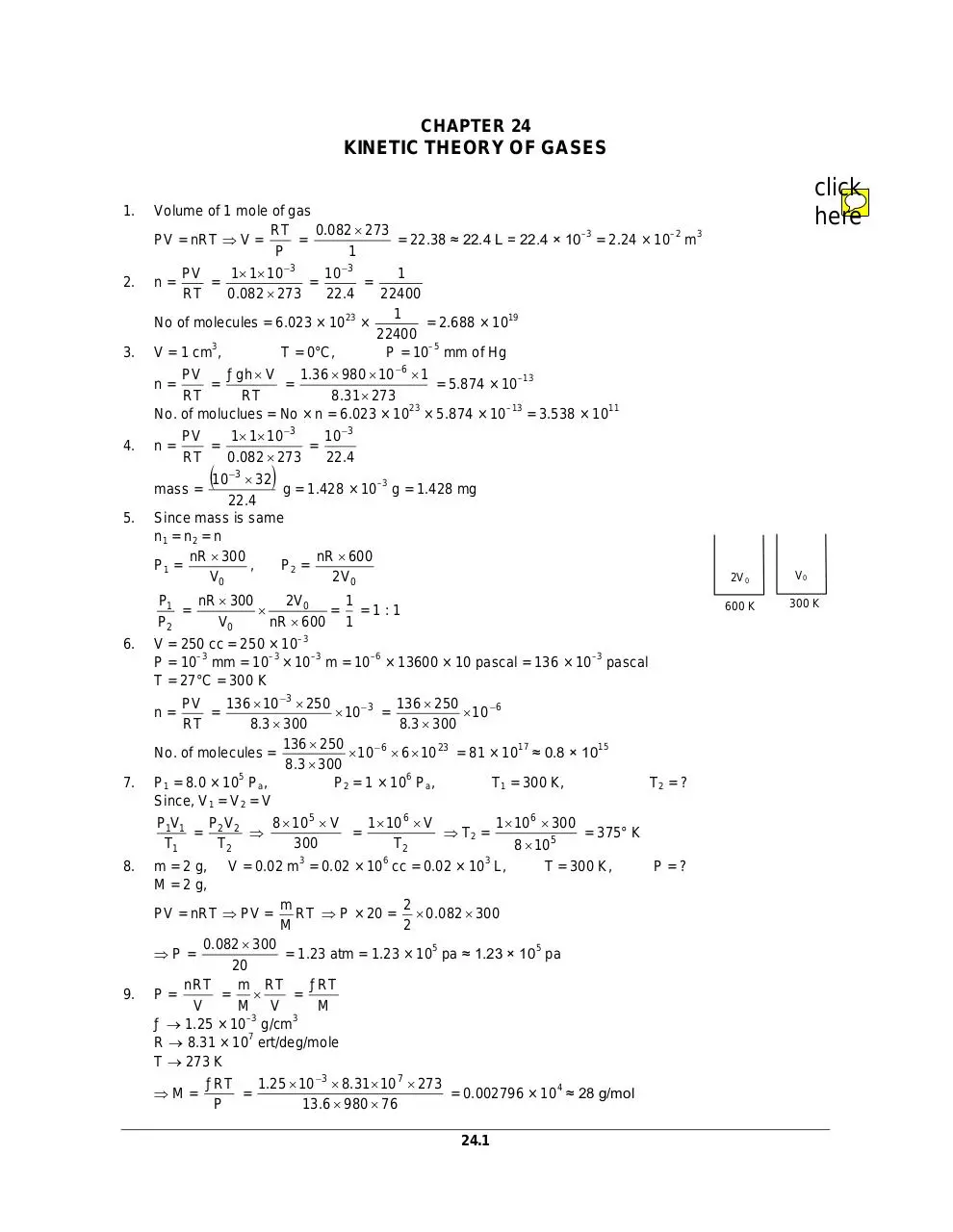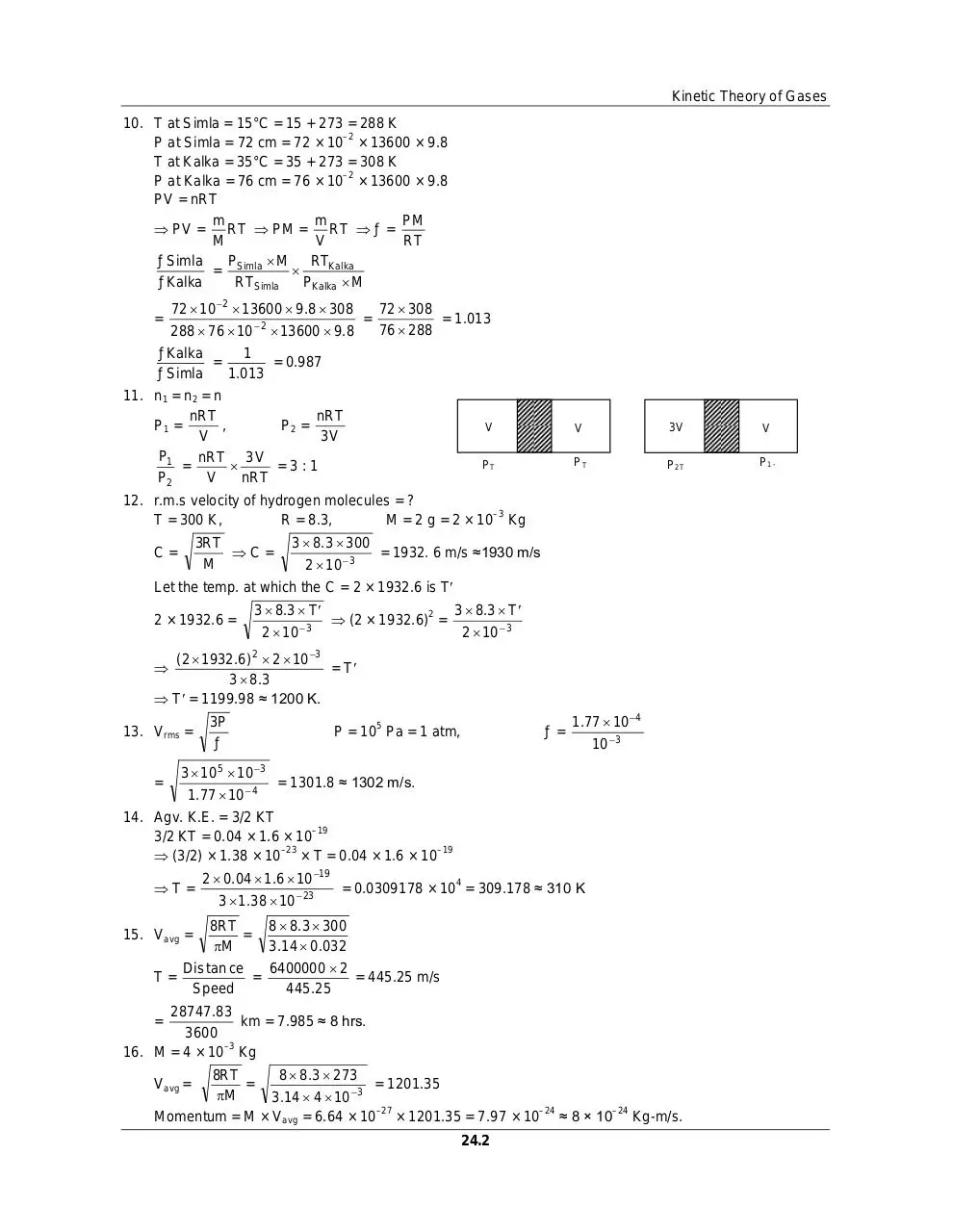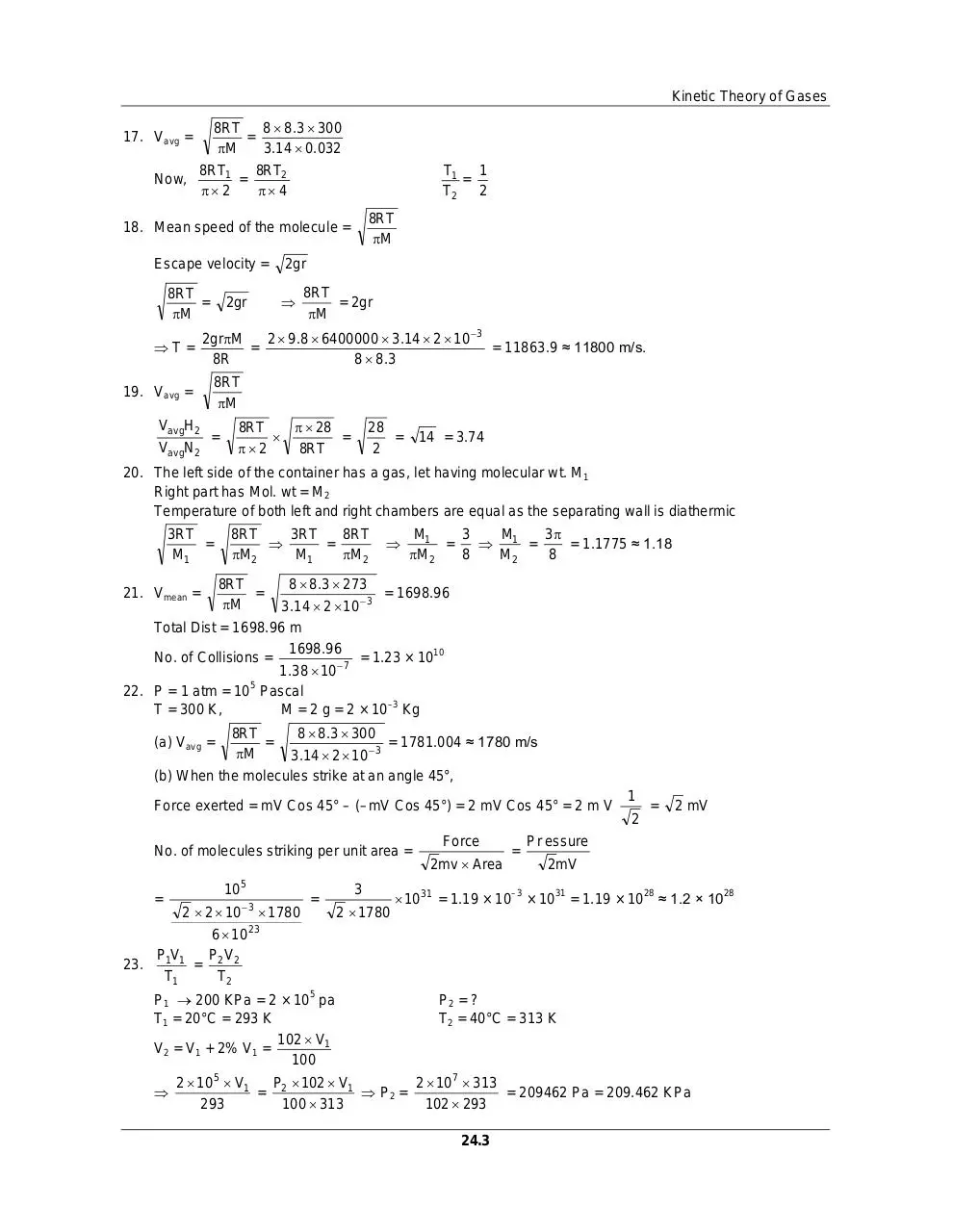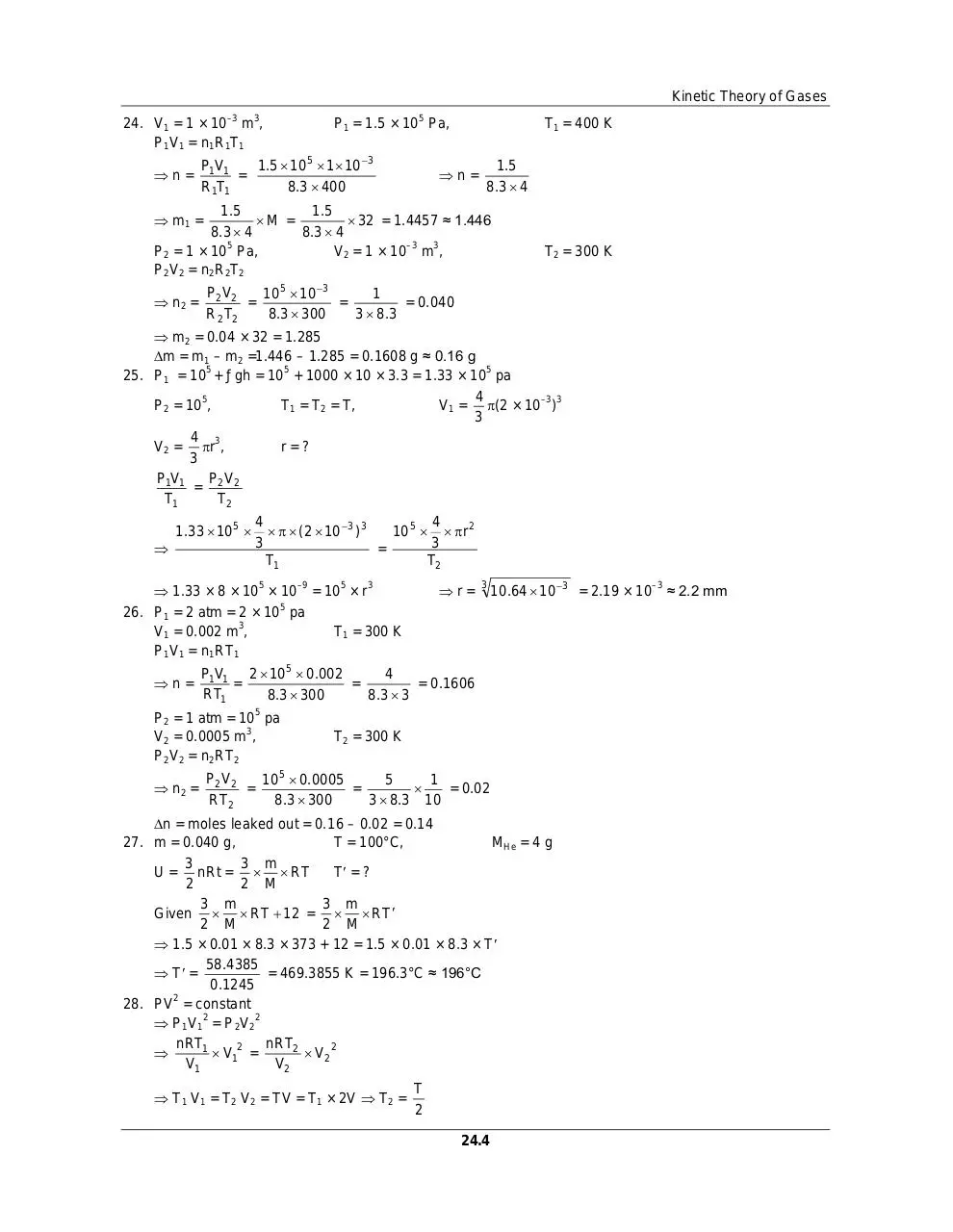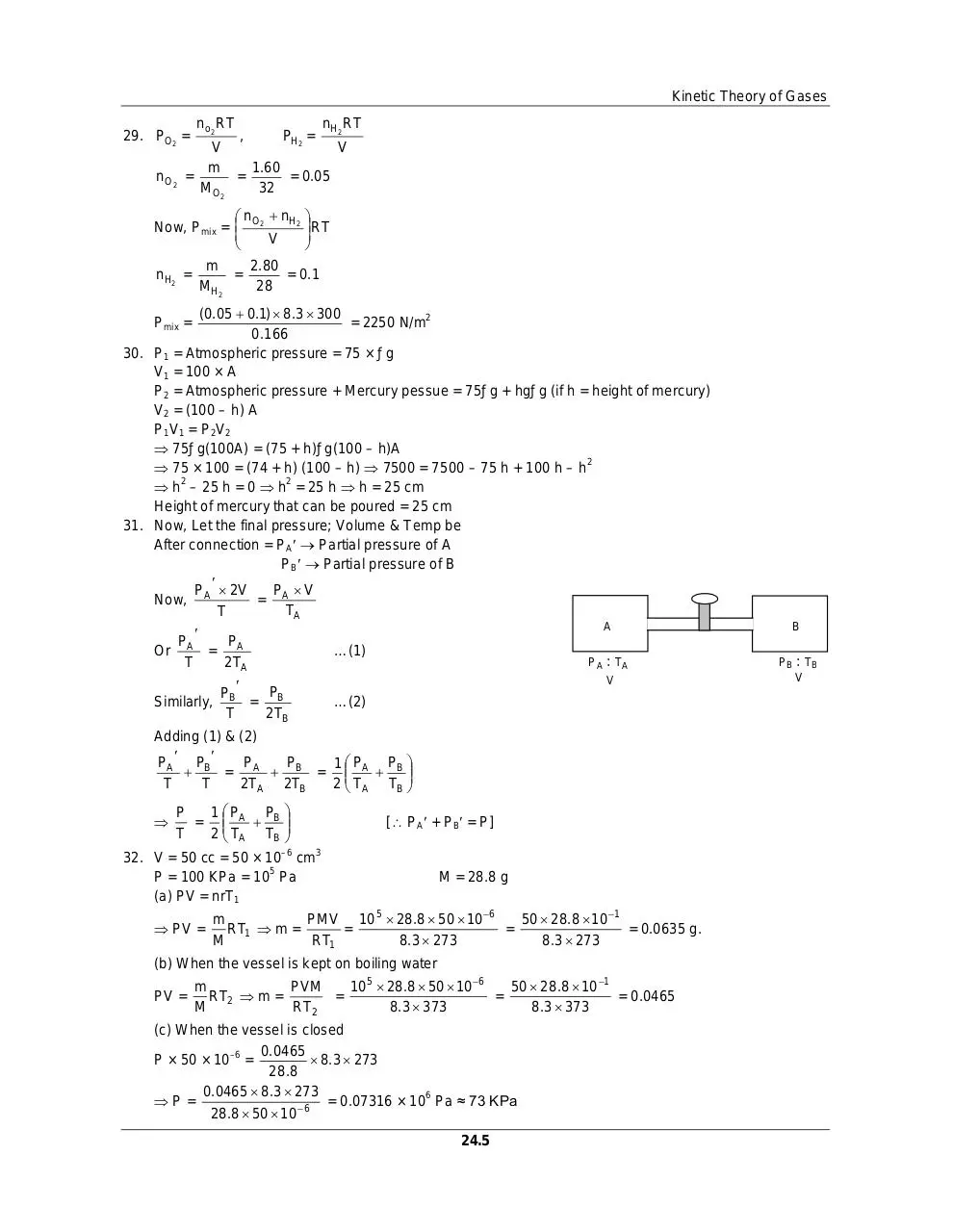H c verma part2 pdf solution.pdf (PDF, 1.96 MB)

### Share on social networks

#### HTML Code

Copy the following HTML code to share your document on a Website or Blog